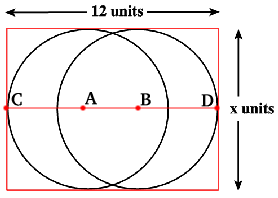You may also likeSquare Pegs

Which is a better fit, a square peg in a round hole or a round peg in a square hole?Cube Paths

Given a 2 by 2 by 2 skeletal cube with one route `down' the cube. How many routes are there from A to B?Coins on a Plate

Points A, B and C are the centres of three circles, each one of which touches the other two. Prove that the perimeter of the triangle ABC is equal to the diameter of the largest circle.

Not So Little X

Age 11 to 14Challenge Level

Congratulations to Matthew Hodgetts, King Edward VI Camp Hill School, Birmingham, to Suzanne Abbott, Nisha Doshi and Christiane Eaves, Mount School, York; to Jessica Zhang; to the KS3 Maths Club, Strabane Grammar School and finally to Michael Swarbrick-Jones, Y7 Comberton Village College, Cambridge whose solution appears below.x = the diameter of one of the circles.
Since (AC) and (BD) are both the length of a radius then x = (AC) + (BD).

So x = 12 - (AB) = 12 - x/3.
So x + x/3 = 12
or 4/3 x = 12.

To find x we take the reciprocal of 4/3 and multiply it by 12.
x = 9 units.